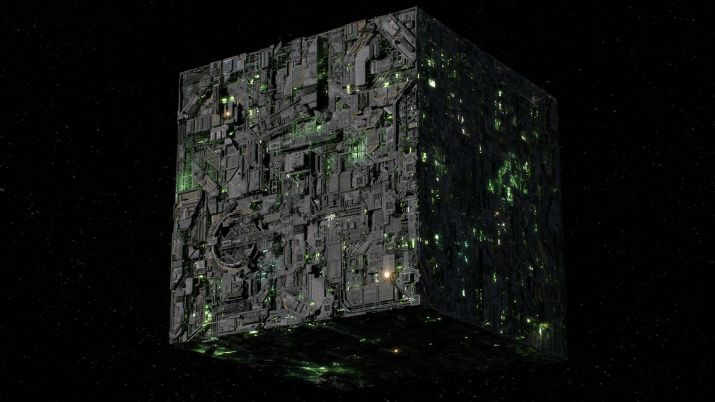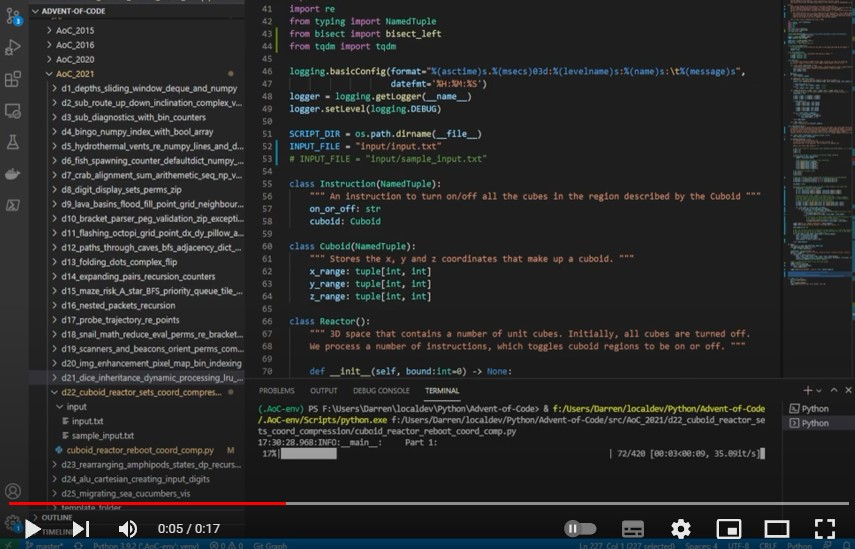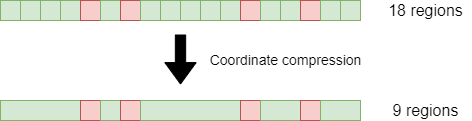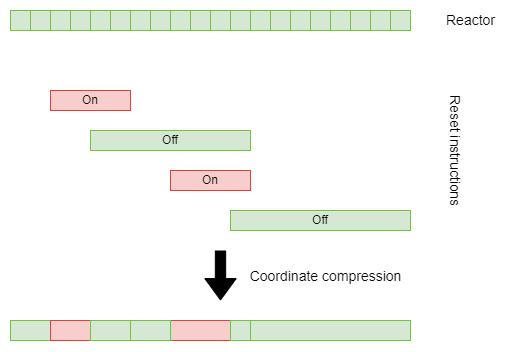# Learning Python with Advent of Code Walkthroughs

Dazbo's Advent of Code solutions, written in Python# Advent of Code 2021 - Day 22

## Problem Intro

I’ve got to admit… At this point in my 2021 AoC journey, I was starting to feel a little grumpy about how much time these challenges were taking. My original expectation was that I’d have most days done by breakfast, and there might be one or two tough days. But at this point, I’m writing off half my annual leave to solving AoC problems. Urgh.

For me, this was one of the toughest challenges. It was hard to get my head around how to solve it. And it was hard to turn my idea into code.

Anyhoo…

We’re told we need to reboot the sub’s reactor. And the reactor is made up of a massive 3D grid of one-unit cubes, where cubes can be `on` or `off`.

We need to follow a reboot sequence, which is a set of `on` or `off` instructions, which each instruction followed by `x`, `y`, and `z` ranges that describe a cuboid. I.e. an `on` instruction will turn all the cubes on, in the 3D cuboid region described by the instruction. And, obviously, an `off` instruction will turn them all off.

Our sample input looks like this:

``````on x=-5..47,y=-31..22,z=-19..33
on x=-44..5,y=-27..21,z=-14..35
on x=-49..-1,y=-11..42,z=-10..38
on x=-20..34,y=-40..6,z=-44..1
off x=26..39,y=40..50,z=-2..11
on x=-41..5,y=-41..6,z=-36..8
off x=-43..-33,y=-45..-28,z=7..25
on x=-33..15,y=-32..19,z=-34..11
off x=35..47,y=-46..-34,z=-11..5
on x=-14..36,y=-6..44,z=-16..29
on x=-57795..-6158,y=29564..72030,z=20435..90618
``````

## Part 1

Considering only cubes in the region `x=-50..50,y=-50..50,z=-50..50`, how many cubes are on after following the reboot sequence?

It’s pretty obvious what Part 2 is going to say. <sigh>

Well, I started off by doing it the obvious way, hoping (foolishly) that it might scale for Part 2.

### Setup

``````from __future__ import annotations
import logging
import os
import time
import re
from typing import NamedTuple
from bisect import bisect_left
from tqdm import tqdm

logging.basicConfig(format="%(asctime)s.%(msecs)03d:%(levelname)s:%(name)s:\t%(message)s",
datefmt='%H:%M:%S')
logger = logging.getLogger(__name__)
logger.setLevel(logging.DEBUG)

SCRIPT_DIR = os.path.dirname(__file__)
INPUT_FILE = "input/input.txt"
# INPUT_FILE = "input/sample_input.txt"
``````

A couple of things we haven’t used before in this AoC:

• `bisect.bisect_left` - a useful package and method for working with sorted lists
• `tqdm` - an awesome package that can turn any loop into a dynamic progress bar!
``````class Instruction(NamedTuple):
""" An instruction to turn on/off all the cubes in the region described by the Cuboid """
on_or_off: str
cuboid: Cuboid

class Cuboid(NamedTuple):
""" Stores the x, y and z coordinates that make up a cuboid. """
x_range: tuple[int, int]
y_range: tuple[int, int]
z_range: tuple[int, int]
``````

Not much to say about that. I’m using a `NamedTuple` for both the `Instruction` class, and for the `Cuboid` class. This just makes them a bit more readable.

Now I’ll create a class that reprents the `Reactor`:

``````class Reactor():
""" 3D space that contains a number of unit cubes. Initially, all cubes are turned off.
We process a number of instructions, which toggles cuboid regions to be on or off. """

def __init__(self, bound:int=0) -> None:
""" Initialise this cuboid set.  When adding / subtracting points (later), ignore anything out of bounds.
Bound is given by (0-bound, 0+bound) in any dimension. 0 means no bound. """
self._bound = bound
self._cuboid = set()

@property
def cubes_on(self):
return len(self._cuboid)

def update(self, instr:Instruction):
""" Turn on / off points that are supplied in the form of a Cuboid. """
cuboid = self._cuboid_to_set(instr.cuboid.x_range, instr.cuboid.y_range, instr.cuboid.z_range)

if instr.on_or_off == "on":
self._cuboid = self._cuboid | cuboid   # union
else:
self._cuboid = self._cuboid - cuboid   # diff

def _cuboid_to_set(self, x_range: tuple, y_range: tuple, z_range: tuple) -> set:
""" Creates a new set of 'on' points, given a set of 3 pairs of cuboid vertices. """
temp_cuboid = set()

x_lower, x_upper = x_range, x_range
y_lower, y_upper = y_range, y_range
z_lower, z_upper = z_range, z_range

if self._bound > 0:
x_lower = max(x_lower, -self._bound)
y_lower = max(y_lower, -self._bound)
z_lower = max(z_lower, -self._bound)

x_upper = min(x_upper, self._bound)
y_upper = min(y_upper, self._bound)
z_upper = min(z_upper, self._bound)

for x in range(x_lower, x_upper+1):
for y in range(y_lower, y_upper+1):
for z in range(z_lower, z_upper+1):

return temp_cuboid

def __repr__(self) -> str:
return f"CuboidGrid:size={len(self._cuboid)}"
``````

• Internally, it stores all `on` cubes as a `set`.
• Thus, the number of cubes turned `on` is given by the size of the `set`. We use the `cubes_on` property to return this value.
• It updates the number of `on` cubes in the reactor, by processing instructions, one instruction at a time.
• For each instruction:

• We create a `set` that contains all the cubes described by the cuboid of this instruction.
• We restrict the cubes in this `set` to be cubes that sit within the bounds, if we passed any. (Which, for Part 1, we did.)
• If we’re turning cubes `on`, we simply `union` all the cubes in the instruction’s cuboid with the cubes that are already on.
• Otherwise, we’re turning the cubes `off`, so subtract all the cubes in the instruction’s cuboid from teh cubes that are already on.

To run it, we first read in all the instructions and split them into lines:

``````input_file = os.path.join(SCRIPT_DIR, INPUT_FILE)
with open(input_file, mode="rt") as f:
``````

Then we convert each line into an `Instruction` object.

``````instructions: list[Instruction] = []
pattern = re.compile(r"(on|off) x=(-?\d+)..(-?\d+),y=(-?\d+)..(-?\d+),z=(-?\d+)..(-?\d+)")
for line in data:
if (match := pattern.match(line)):
instr, x_min, x_max, y_min, y_max, z_min, z_max = match.groups()
reactor = Cuboid((int(x_min), int(x_max)), (int(y_min), int(y_max)), (int(z_min), int(z_max)))
instructions.append(Instruction(instr, reactor))
``````

This works by:

• Using `regex` to obtain capture groups that represent:
• Whether `on` or `off` (`instr`)
• The x start (`x_min`) and x end (`x_max`)
• The y start (`y_min`) and y end (`y_max`)
• The z start (`z_min`) and z end (`z_max`)
• Note that each numeric capture group contains `-?` to allow for optional negative numbers.
• We create a `Cuboid` from the `x`, `y`, and `z` ranges.
• We add the `Instruction` to the `list` of instructions.

Finally, we create our `Reactor` object, with the required bounds, and then process each `Instruction`:

``````# Part 1 - Count how many cubes are on, with small bounds
logger.info("Part 1:")
reactor = Reactor(bound=50)
for instr in tqdm(instructions):
reactor.update(instr)

logger.info("Using CuboidSet - cubes on: %d\n", reactor.cubes_on)
``````

Here I’m using the very cool tqdm package. We use it by wrapping any iterable object. It returns an iterator that behaviours just like the original iterator, but you get a dynamically updating progress bar for free!So that works, and it gives the right answer. Alas, it takes over 10 seconds, which doesn’t bode well for Part 2.

## Part 2

Now we need to run the reboot steps for all cubes in the reactor, not just the ones within the +/-50 ranges.

The instructions tell us that with just the sample data, we end up with 2758514936282235 cubes turned on. That number is just too big to tackle with the same approach. Given that the same sample data only has 547648 `on` cubes for Part 1, even if my computer had enough RAM, and if the application scaled linearly, I’d still be waiting for many years before the program finishes. We’re going to need to be more clever.

My solution is to use coordinate compression. This is a technique where we take a large number of coordinates, and compress them down to fewer coordinates by eliminating all adjacent points where nothing interesting happens. As a really noddy example in one dimension:Here, we’ve taken 18 unit sized regions, which could be represented as `x=0` through to `x=17`. We’ve then compressed the data, by ignoring any x values where nothing changes. This leaves us with only 9 regions that carry any useful information, i.e. where `x` is `0, 4, 5, 6, 7, 12, 13, 15, 16`.

How might we apply this to our reactor problem? Well, let’s start by simplifying our reactor to one dimension. Here’s a simple 1D reactor example:Note how we only mark coordinates where there are instructions that mark the beginning and end of a range. (In my code, I call these ranges intervals.)How does it work?

• Take all the x and y coordinates in the instructions, and assemble all the `x` values in ascending order, and all the `y` values in ascending order. These will be `x` and `y` values where something changes, i.e. either the start of an interval, or the end of an interval.
• The deltas between successive values in each list represent the size of all the intervals, in that particular dimension.
• Thus, by combining `x, y` coordinates and a matching pair of `x` and `y` segment lengths, we are able to obtain all the compressed regions.
• We then iterate through the instructions in instruction order.
• For each instruction, determine the rectangle described by that instruction, in terms of its `x start`, `x end` and `y start`, `y end` coordinates.
• For the pair of `x` values that correspond to the horizontal edges of the rectangle, map them back to index positions.
• Now do the same for `y` values, i.e. corresponding to the vertical edges of the rectangle.
• Now iterate through the `x` indexes from `x1` index to `x2` index, and for each, iterate through the `y` indexes from `y1` index to `y2` index.
• Each loop gives us a segment that is part of an `on` or `off` rectangle.
• So all we need to do is add in `on` segments, and remove `off` segments, as required.

Finally, let’s implement this for Part 2. I’ve created a class called `CuboidDeterminator` to do this:

``````class CuboidDeterminator():
""" Represents number of points that are turned on in a 3D space. This class works using coordinate compression.
Instructions are pre-processed to collapse to coordinates where something changes, i.e. the beginning or end of a cuboid.
We obtain a sorted list of coordinates in each dimension, representing any interval boundaries in that dimension.
For each boundary, we have an associated length of the corresponding cuboid 'segment'.
Finally, we work out how many cubes there are in all the 'on' cuboid segments, and return the total. """

def __init__(self, instructions: list[Instruction]) -> None:
self._instructions = instructions

def perform_reset(self) -> int:
""" Process all the instructions, e.g.
on x=10..12,y=10..12,z=10..12, on x=11..13,y=11..13,z=11..13.
Instruction order is not important.  We'll convert to an ordered list.

Returns int: Total cubes turned on. """

# Here we will store all cube positions where something interesting happens, i.e. a cuboid begins or ends
x_vals = []
y_vals = []
z_vals = []

# Get all the intervals, i.e. where an instruction changes something
for instruction in self._instructions:
cuboid = instruction.cuboid

# Add the intervals (vertices) in this instruction
x_vals.append(cuboid.x_range)
x_vals.append(cuboid.x_range+1)
y_vals.append(cuboid.y_range)
y_vals.append(cuboid.y_range+1)
z_vals.append(cuboid.z_range)
z_vals.append(cuboid.z_range+1)

# All dimensions in numeric order
x_vals.sort()
y_vals.sort()
z_vals.sort()

# Store the intervals (ranges) between each successive value in a given dimension
x_intervals = [x_vals[i+1]-x_vals[i] for i in range(len(x_vals)-1)]
y_intervals = [y_vals[i+1]-y_vals[i] for i in range(len(y_vals)-1)]
z_intervals = [z_vals[i+1]-z_vals[i] for i in range(len(z_vals)-1)]

on_indexes = set()

# Now apply on and off instructions, i.e. add or remove cuboids in the right order
for instruction in tqdm(self._instructions):
# unpack the vertices of this cuboid
x1, x2 = instruction.cuboid.x_range, instruction.cuboid.x_range
y1, y2 = instruction.cuboid.y_range, instruction.cuboid.y_range
z1, z2 = instruction.cuboid.z_range, instruction.cuboid.z_range

# Determine all the cuboid 'segments' we need to turn on.
# A given cuboid in an instruction could contain many smaller cuboid 'segments'.
# Get the indexes for the values given by each instruction, in a given dimension.
# E.g. the first cuboid might give us x1 of 0 and x2 of 2.
# (Because x=1 might be the start of a different cuboid.)
x1_index, x2_index = bisect_left(x_vals, x1), bisect_left(x_vals, (x2+1))
y1_index, y2_index = bisect_left(y_vals, y1), bisect_left(y_vals, (y2+1))
z1_index, z2_index = bisect_left(z_vals, z1), bisect_left(z_vals, (z2+1))

for x_intv_index in range(x1_index, x2_index):
for y_intv_index in range(y1_index, y2_index):
for z_intv_index in range(z1_index, z2_index):
# add starting coords corresponding to cuboid segments to turn on / off
if instruction.on_or_off == "on":
else:
# use discard, to remove cuboid segments that we have previously 'turned on'
# I.e. because an off instruction might overlap.
# If it doesn't overlap, there will be nothing to discard.

logger.info("%d 'on' segments identified.", len(on_indexes))
logger.info("Computing interval volumes. This might take a while...")

total_cubes_on:int = 0

# Each 'on' coord will align to a triplet of interval lengths, i.e. to give the volume of that cuboid 'segment'.
# This gives us the size of the 'on cuboid', i.e. how many cubes are 'on' in the cuboid
# Wrap our set with tqdm to provide a progress bar
for x_intv_index, y_intv_index, z_intv_index in tqdm(on_indexes):
len_x = x_intervals[x_intv_index]
len_y = y_intervals[y_intv_index]
len_z = z_intervals[z_intv_index]
total_cubes_on += len_x * len_y * len_z

``````

The use of `bisect_left` is worthy of a quick mention. Basically, I’m using this to determine the index position of coordinate values in our sorted list of `x`, `y` and `z` values. I’m using this rather than the more usual `x_vals.index(x1)`, because the `index()` method searches for an item sequentally, from beginning to end; whereas the `bisect_left()` method performs a binary search. Performing a binary search is quicker for sorted data. Switching from `index()` to `bisect_left()` shaves about 20 seconds off my program’s overall execution time.

We run it like this:

``````# Part 2 - Count how many cubes are on, with no bounds
logger.info("Part 2:")
reactor = CuboidDeterminator(instructions)
logger.info("Using CuboidDeterminator - cubes on: %d", reactor.perform_reset())
``````

And it follows exactly the same logic as described above, for the 2D example.

It works! Alas, it is a bit slow and takes over 3 minutes to run. So once again, I’m using `tqdm` to generate progress bars.

``````17:49:28.373:INFO:__main__:     Part 1:
100%|███████████████████████████████████████████████████████████████████| 420/420 [00:12<00:00, 33.14it/s]
17:49:41.079:INFO:__main__:     Using CuboidSet - cubes on: 547648
17:49:41.080:INFO:__main__:     Part 2:
100%|███████████████████████████████████████████████████████████████████| 420/420 [02:01<00:00,  3.46it/s]
17:51:42.414:INFO:__main__:     132571629 'on' segments identified.
17:51:42.416:INFO:__main__:     Computing interval volumes. This might take a while...
100%|██████████████████████████████████████████████████| 132571629/132571629 [01:14<00:00, 1790469.93it/s]
17:53:31.546:INFO:__main__:     Using CuboidDeterminator - cubes on: 1206644425246111
17:53:31.547:INFO:__main__:     Execution time: 243.1747 seconds
``````

That’s a flippin’ large number!